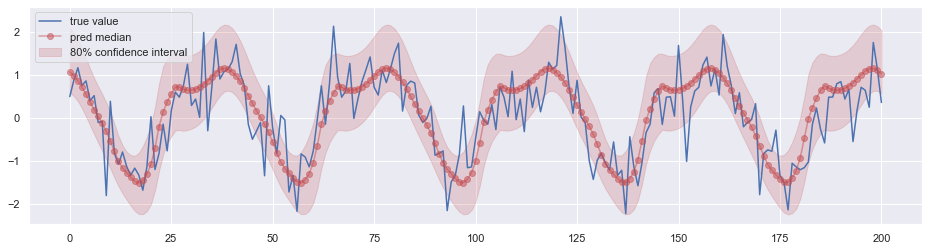$Z$ 表示一个随机变量，其概率密度函数为 $f(z)$，累积分布函数为 $F(z)$。定义函数

$L(Z,\hat Z)=\rho\cdot\max(Z-\hat Z, 0)+(1-\rho)\cdot\max(\hat Z-Z, 0)$

$L(Z,\hat Z)$ 的期望为

\begin{aligned} \mathbb{E}[L(Z,\hat Z)] &= \int_{-\infty}^{+\infty}L(z,\hat Z)f(z)\mathrm dz\\ &= \rho\int_{\hat Z}^{+\infty}(z-\hat Z)f(z)\mathrm dz + (1-\rho)\int_{-\infty}^{\hat Z}(\hat Z - z)f(z)\mathrm dz \end{aligned}

\begin{aligned} \frac{\partial \mathbb{E} [L(Z,\hat Z)]}{\partial \hat Z} &= 0\\ &=-\rho\int_{\hat Z} ^{+\infty}f(z)\mathrm dz+(1-\rho)\int_{-\infty}^{\hat Z}f(z)\mathrm dz\\ &=-\rho[1-F(\hat Z)]+(1-\rho)F(\hat Z)\\ &=F(\hat Z)-\rho \end{aligned}

$\hat Z^*=F^{-1}(\rho)$

DeepAR 等模型中，我们的预测目标是某个确定形式的概率分布的参数，通过最大化对数似然来优化网络。如果我们把预测的目标改为分位数，用 $L(\cdot)$ 作为损失函数呢？下图是实验的结果：